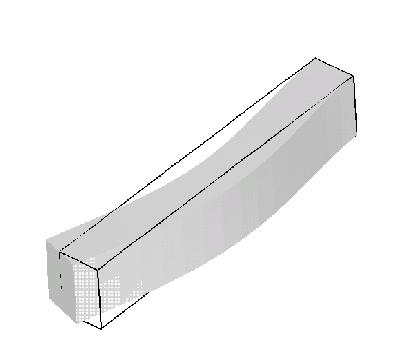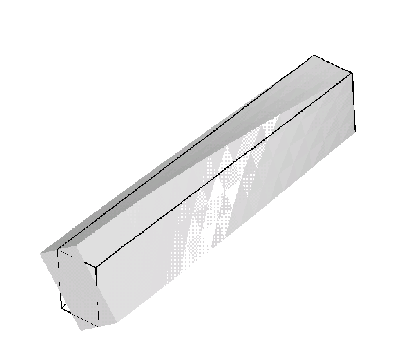## Frequency calculation of a beam loaded by compressive forces

Let us consider the beam from the previous section and determine its eigenfrequencies and eigenmodes. To obtain different frequencies for the lateral directions the cross section is changed from 1x1 to 1x1.5. Its length is kept (8 length units). The input deck is very similar to the one in the previous section (the full deck is part of the test example suite: beamf2.inp):

**
**   Structure: beam under compressive forces.
**   Test objective: Frequency analysis; the forces are that
**                   high that the lowest frequency is nearly
**                   zero, i.e. the buckling load is reached.
**
Model: beam     Date: 10-Mar-1998
*NODE
1,      0.000000,      0.000000,      0.000000
...
*ELEMENT, TYPE=C3D20R
1,     1,    10,    95,    19,    61,   105,   222,   192,     9,    93,
94,    20,   104,   220,   221,   193,    62,   103,   219,   190
...
*BOUNDARY
CN7, 1
*BOUNDARY
CN7, 2
*BOUNDARY
CN7, 3
*ELSET,ELSET=EALL,GENERATE
1,32
*MATERIAL,NAME=EL
*ELASTIC
210000.0,        .3
*DENSITY
7.8E-9
*SOLID SECTION,MATERIAL=EL,ELSET=EALL
*NSET,NSET=LAST
5,
6,
...
*STEP
*STATIC
LAST,3,-48.155
*END STEP
*STEP,PERTURBATION
*FREQUENCY
10
*NODE FILE
U
*EL FILE
S
*END STEP


The only significant differences relate to the steps. In the first step the preload is applied in the form of compressive forces at the end of the beam. In each node belonging to set LAST a compressive force is applied with a value of -48.155 in the positive z-direction, or, which is equivalent, with magnitude 48.155 in the negative z-direction. The second step is a frequency step. By using the parameter PERTURBATION on the *STEP keyword card the user specifies that the deformation and stress from the previous static step should be taken into account in the subsequent frequency calculation. The *FREQUENCY card and the line underneath indicate that this is a modal analysis step and that the 10 lowest eigenfrequencies are to be determined. They are automatically stored in the .dat file. Table 2 shows these eigenfrequencies for the beam without and with preload together with a comparison with ABAQUS (the input deck for the modal analysis without preload is stored in file beamf.inp of the test example suite). One notices that due to the preload the eigenfrequencies drop. This is especially outspoken for the lower frequencies. As a matter of fact, the lowest bending eigenfrequency is so low that buckling will occur. Indeed, one way of determining the buckling load is by increasing the compressive load up to the point that the lowest eigenfrequency is zero. For the present example this means that the buckling load is 21 x 48.155 = 1011.3 force units (the factor 21 stems from the fact that the same load is applied in 21 nodes). An alternative way of determining the buckling load is to use the *BUCKLE keyword card. This is illustrated for the same beam geometry in file beamb.inp of the test suite.

 without preload with preload CalculiX ABAQUS CalculiX ABAQUS 13,096. 13,096. 705. 1,780. 19,320. 19,319. 14,614. 14,822. 76,840. 76,834. 69,731. 70,411. 86,955. 86,954. 86,544. 86,870. 105,964. 105,956. 101,291. 102,148. 162,999. 162,998. 162,209. 163,668. 197,645. 197,540. 191,581. 193,065. 256,161. 256,029. 251,858. 253,603. 261,140. 261,086. 259,905. 260,837. 351,862. 351,197. 345,729. 347,688.

Figures 8 and 9 show the deformation of the second bending mode across the minor axis of inertia and deformation of the first torsion mode.